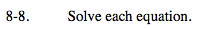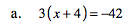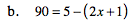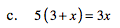### Home > MC2 > Chapter 8 > Lesson 8.1.1 > Problem8-8

8-8.Distribute.
3(x) + 3(4) = −42

Simplify.
3x + 12 = −42

Subtract 12 from each side.
3x + 12 = −42
−12 −12
3x = −54

x = −18

Distribute.
90 = 5 − 2x − 1

Simplify.
90 = 4 − 2x

Subtract 4 from each side.
90 = 4 − 2x
−4 −4
86 = −2x

x = −43

Follow the methods presented in part (a) and part (b).